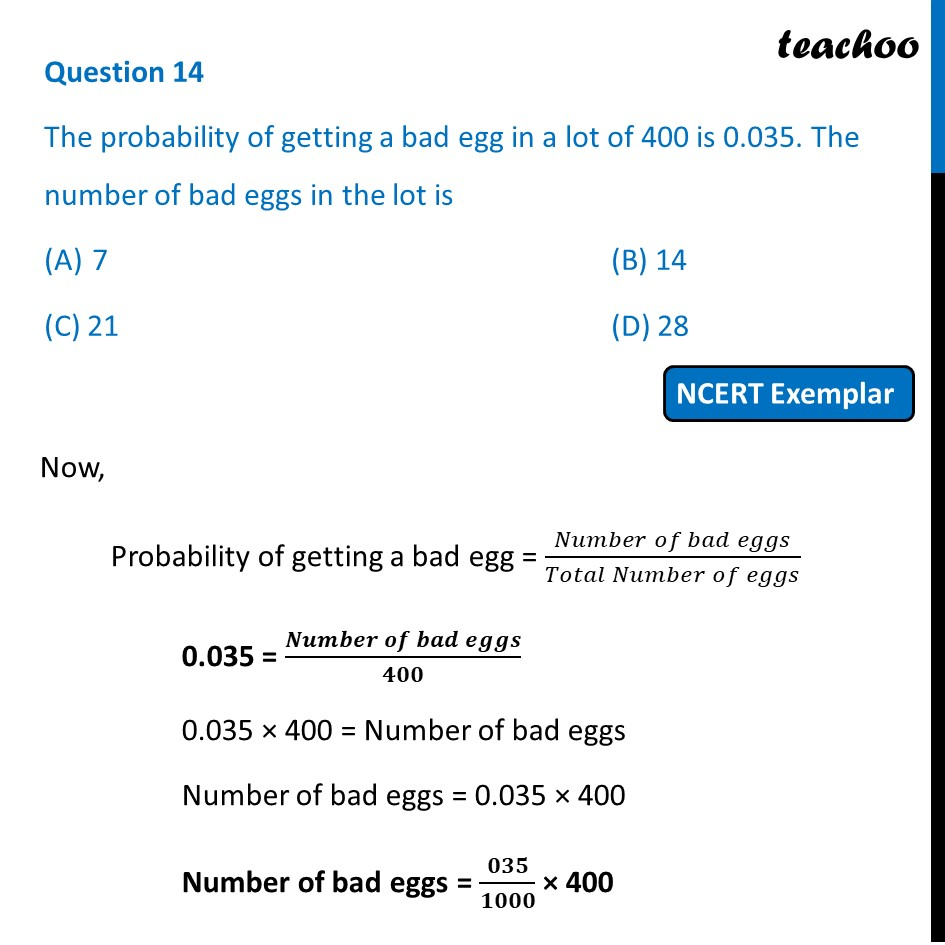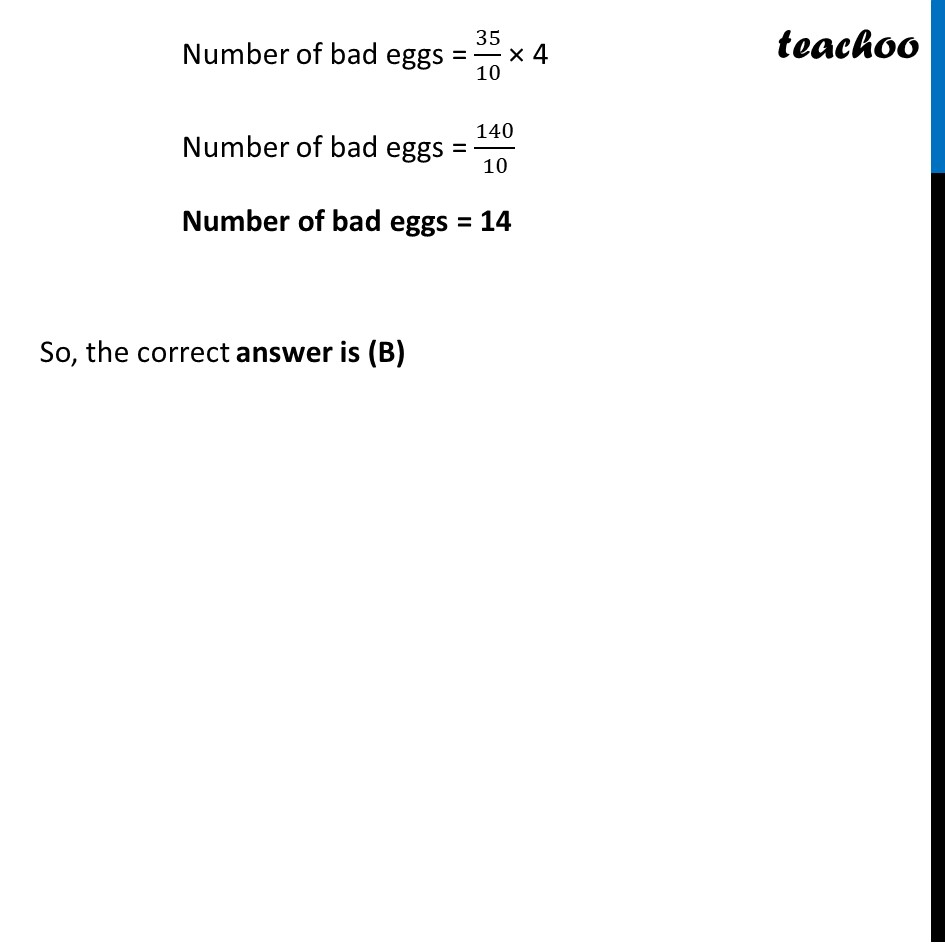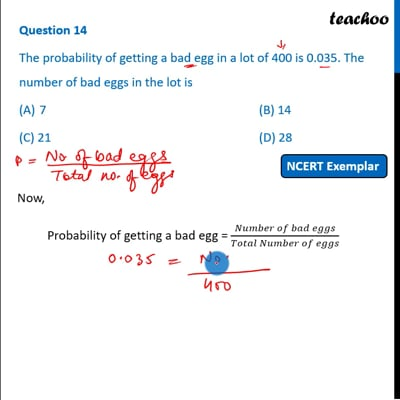NCERT Exemplar - MCQ

Chapter 15 Class 10 Probability
Serial order wise

## (C) 21   (D) 28This video is only available for Teachoo black users

Maths Crash Course - Live lectures + all videos + Real time Doubt solving!

### Transcript

Question 14 The probability of getting a bad egg in a lot of 400 is 0.035. The number of bad eggs in the lot is 7 (B) 14 (C) 21 (D) 28 Now, Probability of getting a bad egg = (𝑁𝑢𝑚𝑏𝑒𝑟 𝑜𝑓 𝑏𝑎𝑑 𝑒𝑔𝑔𝑠)/(𝑇𝑜𝑡𝑎𝑙 𝑁𝑢𝑚𝑏𝑒𝑟 𝑜𝑓 𝑒𝑔𝑔𝑠) 0.035 = (𝑵𝒖𝒎𝒃𝒆𝒓 𝒐𝒇 𝒃𝒂𝒅 𝒆𝒈𝒈𝒔)/𝟒𝟎𝟎 0.035 × 400 = Number of bad eggs Number of bad eggs = 0.035 × 400 Number of bad eggs = 𝟎𝟑𝟓/𝟏𝟎𝟎𝟎 × 400 Number of bad eggs = 35/10 × 4 Number of bad eggs = 140/10 Number of bad eggs = 14 So, the correct answer is (B)